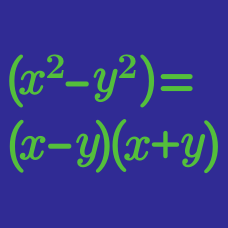Algebra

# Factoring Polynomials

Completely factorize $x^3-3x^2+4x-12.$

Find the sum of all real solutions of the equation $x^2-36=3(x-6)^2.$

Completely factorize $x^4+15x^3+70x^2+120x+64.$

If $$f(x)=x^3-(3+a)x^2+(2+3a)x-2a$$ and $$g(x)=x^3+4x^2+8x+8$$ share a common factor, what is the value of $$a?$$

If the complete factorization of $$x^3-1x^2-26x-24$$ gives $(x+a)(x+b)(x+c),$ what is the value of $$abc?$$

×

Problem Loading...

Note Loading...

Set Loading...